HELLO, GUEST## Tower of Hanoi – Solutions

Introduction

The Tower of Hanoi is a puzzle popularized in 1883 by Edouard Lucas, a French scientist famous for his study of the Fibonacci sequence. However, this puzzle’s roots are from an ancient legend of a Hindu temple. The legend states that there is a secret room in a hidden temple that contains three large pegs. One of these poles has 64 golden disks stacked upon it, each disk being smaller then the disk underneath it, with the biggest disk at the bottom. Since the beginning of time, monks have been trying to shift the 64 disks onto the third peg. The monks also can only transfer the disks if the following rules are followed. First, the monks can only move one disk at a time, and second, they cannot put larger disks on top of smaller disks. Once all 64 disks are shifted to the third peg, the world will end. After encountering this puzzle (in a simpler form) at a science fair a couple years ago and seeing it occasionally even today in an engineering classroom environment, I decided that this was the perfect opportunity to examine this puzzle at a deeper level.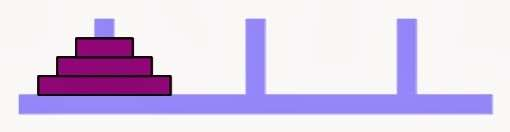(A basic rendition of a 3 disk tower)

Aim

My aim is to explore the different patterns that lead to the answer to the legend: how much time would it take for the world to end?

Finding an Optimal Strategy

In order to get closer to solving this puzzle, the goal will be to find the most efficient way to get 64 disks onto the third peg. To better grasp the mathematical concepts and patterns when solving the tower, it would be easier to look at a simpler version of the puzzle, such as the following 3 disk example.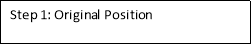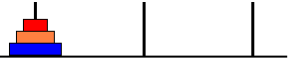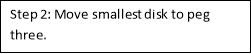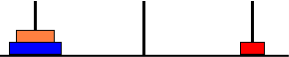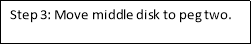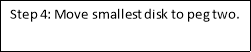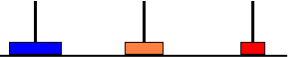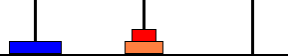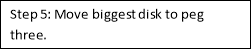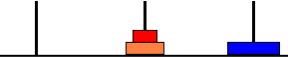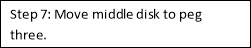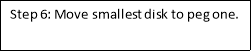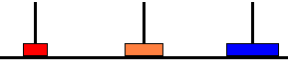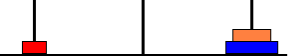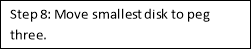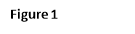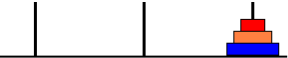Binary Code (Standard Gray Code)

We can relate the pattern seen above to binary code, specifically Standard Gray Code. Standard Gray Code is a binary numeral system where two successive values differ in only one (binary) digit. Using this method may bring us closer to being able to solve the 64 disk tower. If we relate the example in Figure 1 with Standard Gray Code, using 3 binary digits, we are left with something like this:

 000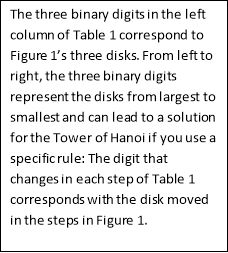Step 1 001 Step 2 011 Step 3 010 Step 4 110 Step 5 111 Step 6 101 Step 7 100 Step 8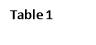For example, Step 1 is shown by the three digits 000. The next step is 001, changing the digit that corresponds with the smallest disk, which means disk 1 is the first disk to move in the solution. And to continue, Step 2 is 011, showing that now the middle (second) disk is being moved. This method could lead us to the solution of a 64 disk tower, as it would show which disk to move; however, the flaw in this method is that even though the binary digits can show which disk has moved, it does not show where to move it. There are always two possibilities for each disk, and when we factor in the 64 other disks, the calculations get extremely tedious and suboptimal as a solution.

Recursive Pattern

The next viable solution is finding a recursive pattern to determine how many moves it would take to solve the puzzle, depending on the number of disks. A recursive pattern uses information from the previous step to find the next. In order to move n amount of disks from peg 1 to peg 3, we can again refer to Figure 1. The first step is transferring n-1 disks from peg 1 to peg 3. We assign a variable to the number of moves this takes, in this case, M. Next, transfer the middle disk to peg 2 (step 3) and finally, transfer the remaining disks from peg 3 to peg 2 (step 4). When you move n amount of disks to any peg, the number of moves will be the same, no matter which direction you choose to go.

If you need assistance with writing your essay, our professional essay writing service is here to help!

From this, we can find an equation to finding the moves needed for any number of disks:          2M + 1, where M equals the number of moves needed to transfer n-1 disks from peg 1 to peg 2.

This brings up another flaw to the problem. In order to find how many moves needed to transfer 64 disks, we also need to calculate the number of moves for 63, 62, 61, etc amount of disks as well. Because of this, the recursive pattern cannot be used to find the time it takes before the world ends. However, what the recursive pattern can do is generate numbers that lead into a non recursive pattern.

 # of Disks # of Moves 2M + 1 1 1 2(0) + 1 = 1 2 3 2(1) + 1 = 3 3 7 2(3) + 1 = 7 4 15 2(7) + 1 = 15 5 31 2(15) + 1 = 31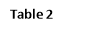From Table 2, we can see that the third column represents a geometric progression that can help us find a formula for a non-recursive pattern.

Non-Recursive Pattern (Explicit Pattern)

When looking back at Table 2, there is a direct correlation that can be made from the number of disks and the number of moves. Recognizing that there is a geometric progression, one could infer the pattern that is being used though the power of two.

 # of Disks # of Moves 1 21 – 1 = 1 2 22 – 1 = 3 3 23 – 1 = 7 4 24 – 1 =15 5 25 – 1 =31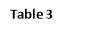Therefore the function to find the number of steps with any number of disks would be 2– 1, with n being the # of disks.

Just to further prove that 2n – 1 is the correct function, we can graph 2n – 1 and compare to the number of disks and moves in Table 2.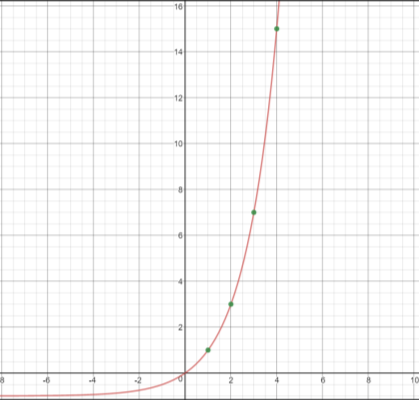It completely fits the data points, confirming the relation between the points and the function. Now we can just plug in the function: 264 – 1 = 590,000,000,000 years

Conclusion

In order to move 64 disks from the first peg to the third, the monks would need over 590 billion years, assuming that they can move one disk per second. The function 2n – 1 was found by recognizing the geometric progressions in the recursive formula and using it in an explicit pattern. This function can be used to find the most optimal number of moves it would take to move any number of disks to the third peg.

Bibliography

Bogomolny, Alexander. “Tower of Hanoi.” Tower of Hanoi. N.p., n.d. Web. 3 Mar. 2017. <http://www.cut-the-knot.org/recurrence/hanoi.shtml>.

Johnson, P. Sam, Recurrence Relations And Their Solutions (Problem : Tower Of Hanoi), 2015 December 26, and 1/1. “Information on Subsets of a Set.” N.p., n.d. Web. 3 Mar. 2017. <theory.cs.uvic.ca/inf/comb/SubsetInfo.html#Hanoi>.

Longman, Addison Wesley. “Miller’s Mathematical Ideas, 9th Edition Web Site Chapter 4 — Internet Project.” Miller’s Mathematical Ideas, 9th Edition Web Site Chapter 4 — Internet Project. Pearson Education, n.d. Web. 3 Mar. 2017.

Math, Dr. “Ask Dr. Math FAQ: Tower of Hanoi.” Mathforum.org. Drexel University, n.d.   Web. 3 Mar. 2017.## Most Used Categories

Recommendation
With Our Resume Writing Help, You Will Land Your Dream Job
Resume Writing Service, Resume101
Trust your assignments to an essay writing service with the fastest delivery time and fully original content.
Essay Writing Service, EssayPro
Nowadays, the PaperHelp website is a place where you can easily find fast and effective solutions to virtually all academic needs
Universal Writing Solution, PaperHelp
Professional Custom
Professional Custom Essay Writing Services
In need of qualified essay help online or professional assistance with your research paper?
Browsing the web for a reliable custom writing service to give you a hand with college assignment?
Out of time and require quick and moreover effective support with your term paper or dissertation?